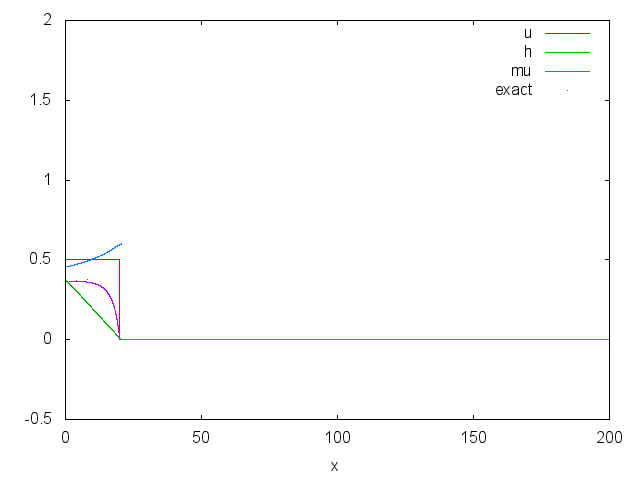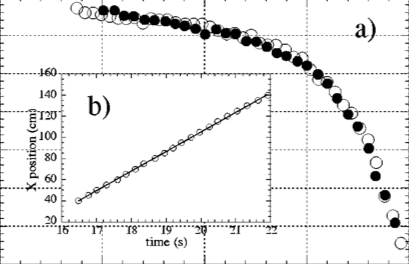# Shape of a granular front down a rough inclined plane,

## Problem

An long avalanche is flowing along a mild slope, what is the shape of this avalanche, and how is the front of the avalanche.

This is Pouliquen’s 99 problem.

Gif animated of the propagation of the front (reload to refresh) or click on image for animation:Images of the front## Equations

Simulation in 1D of the fundamental experiments by Pouliquen 99 revisited by the longitudinal viscosity by Edwards & Gray 14

We solve the Savage-Hutter-Depth-Averaged-Shallow-Water-Saint-Venant equations in 1D

\displaystyle \frac{\partial }{\partial t} h \; +\; \frac{\partial }{\partial x} uh=0 \displaystyle \frac{\partial }{\partial t} hu + \frac{\partial }{\partial x} \dfrac{(hu)^2}{h} + \frac{\partial }{\partial x}g\dfrac{h^2}{2} = - gh \frac{\partial }{\partial x} Z-\mu(I) g h\frac{u}{|u|} + \frac{\partial }{\partial x}(\nu_e h^{3/2}\frac{\partial }{\partial x}u) over a rough inclined plane Z=-\tan \theta x with the \mu friction law \displaystyle \mu=\mu_0 + \frac{ \Delta \mu}{1+I_0/I} where I=\frac{5 (d_g)|u|}{2\sqrt{gh}h} is the mean inertial/ Froude / Savage number, where d_g is the grain diameter. Note that the problem is without dimension, if space is measured by H, the speeds are measured by \sqrt{g H} (see remark on t). So, there is no g and \rho in I. Note that in the fundamental papers of Pouliquen, the function \mu is different (and the value of the parameters of the friction law as well).

An extra viscous term has been added by Edwards and Gray corresponding to a longitudinal viscosity depending on the height. This new viscous term \nu_e h^{3/2} will be explained later.

## Code

#include "grid/multigrid1D.h"
#include "saint-venant.h"

The problem is solved in one dimension, but can be extended to two.

double u0,h0;
double mu0,Deltamu,I0,dg;
double Ltas,tmax,tantheta,nue,Itheta;

Wall symmetry at the left Neumann conditions at the exit. imposed velocity: velocity of all the moving avalanche Neuman on the height (the sin is to add roll waves for fun)

u.n[left] = u0*(1.+.1*0*sin(t/3));
h[left] =  neumann(0);
u.n[right] =  neumann(0);
h[right] = neumann(0);//h[];

Main and parameters

int main()
{

The domain is 100 long, tantheta is \tan \theta, Initial shape and velocity

  X0 = 0.;
L0 = 200;
tantheta = 0.3838640;//tan(21*pi/180
Ltas = 20;
u0 = .5;

parameters for the fiction law let use H as scale, diameter of the grain is more or less 1./20 the unit

  dg = .5/11.6;
mu0 = 0.35;
Deltamu = .21;
I0 = 0.4;

let use H as scale, taking G=1, means that velocity is measured by \sqrt(gH), a good practical idea is to take H=1cm.

  G = 1.;

need a very small step for precision dx = 100/(16*1024.) = 0.006

  N = 1024*4*2;
tmax = 200-50;

The value of I corresponding to the angle \theta is: \displaystyle I_ \theta= I_0(\tan \theta-\mu_0)/(\mu_0+\Delta \mu-\tan \theta)

   Itheta = I0*(tantheta-mu0)/(mu0+Deltamu-tantheta);

the Bagnold mean velocity at this value of \theta as a function of the hight, and the height as function of the mean velocity are then: \displaystyle u_0 = \frac{2 I_{\theta}}{5} \sqrt{g h_0} \frac{h_0}{d_g}\;\;\; h0 = ((5./2)/I_{\theta}/\sqrt(g) u_0 d_g)^{ 2/3}

//   u0 = (2./5)*Itheta*sqrt(G*h0)*h0/dg;
h0 = pow( (5./2)/Itheta/sqrt(G)*u0*dg , 2./3);

Edwards and Gray 14 proposed a viscosity comming from the \partial_x \tau_{xx} term, through integration, this gives \displaystyle \frac{\partial }{\partial x}(\nu_e h^{3/2}\frac{\partial }{\partial x}u) where $e=5 d sin /(9 I)$ that we approximate as

  nue =  5*dg/(9*Itheta)*tantheta;
fprintf (stderr," u0=%lf  h0=%lf Ia=%lf  nu=%lf\n",u0,h0,Itheta,nue);

If viscosity \nu_e is not zero, the maximal time step is defined due to this viscosity:

//  DT = (L0/N)*(L0/N)/2/nue;
run();
}

The initial conditions

event init (i = 0){

the inclined plane is the topography

   foreach(){
zb[] =  -tantheta*x;}

Initial distribution of materials a slope and a constant velocity

   foreach(){
h[]= (x < Ltas) ?  h0 *(1-x/Ltas): 0;
u.x[]= (x < Ltas) ?  u0 *(1+.0*sin(x/9)): 0;}
boundary ({u.x,h});
}
event coulomb_friction (i++) {
double In,mu,ff;

We use a simple implicit scheme to implement coulomb bottom friction i.e. (note the simplification by h) \displaystyle \frac{d\mathbf{u}}{dt} = -\mu g \frac{\mathbf{u}}{|\mathbf{u}|} with \mu fonction of I. Note that the good implementation preserving equilibrium balance is in Bouchut’s book

  foreach() {
In=(dg)*5./2.*norm(u)/pow(h[],1.5)/sqrt(G);
mu = (mu0 + Deltamu*In/(I0+In)) ;
//  mu = (0.4 + 0.26*exp(-0.136/In));
ff = norm(u) > 0 ? (1. +  dt *mu*G/(norm(u))) : HUGE ;

foreach_dimension()
u.x[] /= ff ;
}
boundary ({u.x,h});
}

The new viscous term from Gray & Edwards \displaystyle \frac{\partial }{\partial x}(\nu_e h^{3/2}\frac{\partial }{\partial x}u)

/*
event friction_long (i++) {
foreach_dimension() {
face vector g[];
scalar a = u.x;
foreach_face()
g.x[] = nue*(a[] - a[-1,0])/Delta*pow((h[0,0] + h[-1,0])/2,3./2);
foreach ()
u.x[] += dt/Delta*(g.x[1,0] - g.x[] + g.y[0,1] - g.y[]);
}
boundary ((scalar *){u});
}
*/

output

event output (t += 1; t <= tmax) {
double In,ut,ht,xf=0;

tracking the front

  foreach()
xf = h[] > dry ?  max(xf,x) :  xf ;

monitoring the values at the middle of the avalanche

  ut=interpolate(u.x,u0*t/2,0);
ht=interpolate(h,u0*t/2,0);
In=5./2.*dg*ut/ht/sqrt(G*ht);
fprintf (stderr," t= %lf  u=%lf  h=%lf I=%lf mu(I)=%lf Fr= %lf xf = %lf \n",
t,ut,ht,In,(mu0 + Deltamu*In/(I0+In)),5./2.*ut/sqrt(G*ht),xf);

For the steady established avalanche, we have the value of I for the angle \theta: \displaystyle I_ \theta= I_0(\tan \theta-\mu_0)/(\mu_0+\Delta \mu-\tan \theta) and the Bagnold mean velocity associated to this value of the angle \displaystyle u_0 = \frac{2 I_{ \theta}}{5} \sqrt{g h_0} \frac{h_0}{d_g}

We want to see how the front connects a region with no flow, to a region with the established Bagnold profile. The exact solution for the front propagation, there exists a moving solution at constant velocity u_0: h(x-u_0 t), the mass conservation is satisfied \displaystyle -u_0\frac{\partial }{\partial x} h \; +\; \frac{\partial }{\partial x} uh=0 so that u=u_0 the velocity is everywhere constant: \displaystyle - u_0^2 \frac{\partial }{\partial x} h + u_0^2 \frac{\partial }{\partial x} h + \frac{\partial }{\partial x}g\dfrac{h^2}{2} = - gh \frac{\partial }{\partial x} Z-\mu(I) g h\frac{u}{|u|} +0 we then have an equilibrium of the pressure terms and the friction terms in the SH equation, which gives \displaystyle \frac{dh }{dx} = (\tan \theta - \mu_0) - \frac{\Delta \mu}{1 + I_0/I} there exists a solution if the velocity is constant, so replacing I_0/I by its value, which gives I_0/I=(h/h_0)^{3/2} \frac{\Delta \mu - (\tan \theta - \mu_0)}{(\tan \theta - \mu_0)}. Note that I_0 desappears.

We define H=h/H_0, and X=x (\tan \theta - \mu_0)/h_0 and d= (\tan \theta - \mu_0)/\Delta \mu, so we have without dimension \displaystyle \frac{dH}{dX} = (1 - \frac{1}{d + H^{3/2} (1-d)}) we can integrate it. We can solve the inverse \frac{dX}{dH} which gives the position as a function of the hight: \displaystyle X= \frac{(d-1) H-\frac{2}{3} \log \left(1-\sqrt{H}\right)+\frac{1}{3} \log \left(H+\sqrt{H}+1\right)-\frac{2 \tan ^{-1}\left(\frac{2 \sqrt{H}+1}{\sqrt{3}}\right)}{\sqrt{3}}}{d-1}

Thanks to the fact that the solution is at constant velocity, this solution does not depend on \nu_e.

#ifdef gnuX

the variable gnuX is passed trough the run, when it is set, the output is transfered by a pipe to gnuplot

  fprintf (stdout,"set xlabel 'x'; mu(x)=%lf+%lf*x/(%lf+x) \n",mu0,Deltamu,I0);
fprintf (stdout,"xdeh(h)=((-1+d)*h-(2*atan((1+2*sqrt(h))/sqrt(3)))/sqrt(3)-(2*log(1 - sqrt(h)))/3.+log(1+sqrt(h)+h)/3.)/(-1+d) \n");
fprintf (stdout," d= %lf \n",(tantheta-mu0)/Deltamu);
fprintf (stdout,"xdeh0(h)=(xdeh(h/%lf)-xdeh(0))/%lf*%lf + %lf \n",h0,(tantheta-mu0),h0,xf);
fprintf (stdout,"p[0:%lf][-.5:2]'-'u ($1):2 t'u' w l\ ,'-' u ($1):3 t'h' w l,'-' u ($1):(mu((%lf*5./2.*$2/($3**1.5)))) t'mu' w l,'-' u (xdeh0($3)):3 t'exact' w d \n",L0,dg);

foreach()
fprintf (stdout, "%g %g %g  \n", x, u.x[], h[]);
fprintf (stdout, "e\n\n");
#else

standard output, i.e. as on the web

  foreach()
fprintf (stdout, "%g %g %g \n", x-xf, h[], t);
fprintf (stdout, "\n");
#endif

save the front

  ht=interpolate(h,u0*t/10,0);
FILE *  f = fopen("front.txt", "w");
foreach()
fprintf (f, "%g %g \n", fmin((x-xf)/ht,0), h[]/ht);
fclose(f);

FILE *  g = fopen("frontgnu.gnu", "w") ;
fprintf (g,"set xlabel 'x'; mu(x)=%lf+%lf*x/(%lf+x) \n",mu0,Deltamu,I0);
fprintf (g,"xdeh(h)=((-1+d)*h-(2*atan((1+2*sqrt(h))/sqrt(3)))/sqrt(3)-(2*log(1 - sqrt(h)))/3.+log(1+sqrt(h)+h)/3.)/(-1+d) \n");
fprintf (g," d= %lf \n",(tantheta-mu0)/Deltamu);
fprintf (g,"xdeh0(h)=(xdeh(h/%lf)-xdeh(0))/%lf*%lf + %lf \n",h0,(tantheta-mu0),h0,xf);
fclose(g);
}

# Run

To run and plot with gnuplot through a pipe

qcc -g -O2 -DTRASH=1 -Wall -DgnuX=1 -o front_poul_ed front_poul_ed.c -lm
./front_poul_ed | gnuplot 

To run and make a film (a gif animated)

 qcc -g -O2 -DTRASH=1 -Wall -DgnuX=1 -o front_poul_ed front_poul_ed.c -lm
./front_poul_ed > v.out
echo "set term gif animate; set output 'front_poul_ed.gif'" > dmp
cat v.out >> dmp
mv dmp v.out
cat v.out | gnuplot
  gifsicle --colors 256 --optimize --delay 1   --loopcount=0 front_poul_ed.gif > front_poul_edo.gif 

To run like on the web

qcc -g -O2 -DTRASH=1 -Wall   -o front_poul_ed front_poul_ed.c -lm
./front_poul_ed > out 

## Results

velocity

Profiles for t>150 from numerics

set xlabel "x-xf"
set ylabel "h(x,t)"
p'out' u ($1<0 ? ($3 > 150 ? $1 : 0) : 0):($3 > 150 ? $2:0)shape of front from the out file (script) Compare final profile from numerics with Pouliquen’s experiment reset X0=356 X1=85 Y0=48 Y1=190 unset tics p[0:][0:]'../Img/front_poul_ed_4_21.png' binary filetype=png with rgbimage not,'front.txt'u (X0+($1/60)*(X0-X1)):(($1>-60)?Y0+$2*(Y1-Y0):NaN) t 'comp' w lpcompare with Pouliquen’s experiment (script)

We see how the shape changes if the parameters are changed

reset
set xlabel "x-xf"
set ylabel "h(x,t)"
xdeh(h,d,dmu)=(((-1+d)*h-(2*atan((1+2*sqrt(h))/sqrt(3)))/sqrt(3)-(2*log(1 - sqrt(h)))/3.+log(1+sqrt(h)+h)/3.)/(-1+d)-(-(2*atan((1 )/sqrt(3)))/sqrt(3)-(2*log(1))/3.+log(1)/3.)/(-1+d))/(d*dmu)

mu0 = 0.35
Deltamu = .21
tantheta = 0.383864
set key bottom left

p[-60:2][]'front.txt'  t'num' w l,'' u (xdeh($2,(tantheta-mu0)/Deltamu,Deltamu)):2 t'exact' w l,'' u (xdeh($2,(tantheta-mu0)/1.5/Deltamu,1.5*Deltamu)):2 t'increase Delta mu'w l,'' u (xdeh($2,(tantheta-mu0*1.025)/Deltamu,Deltamu)):2 t'increase mu'w l,'' u (xdeh($2,(tantheta*1.05-mu0)/Deltamu,Deltamu)):2  t'increase theta'w lshape of front, sensibility to variations of parameters (script)

Version 1 Séville 10/2014 - Tunis 11/2014

• savagestaron.c